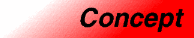# UniqueHashedAssociativeContainerCategory: containers Component type: concept

## Description

A Unique Hashed Associative Container is a Hashed Associative Container that is also a UniqueAssociativeContainer. That is, it is a HashedAssociativeContainer with the property that no two elements in the container have the same key.

## Associated types

None, except for those described in the HashedAssociativeContainer and UniqueAssociativeContainer requirements.

## Notation

 `X` A type that is a model of Hashed Associative Container `a` Object of type `X` `t` Object of type `X::value_type` `k` Object of type `X::key_type` `p`, `q` Object of type `X::iterator` `n` Object of type `X::size_type` `h` Object of type `X::hasher` `c` Object of type `X::key_equal`

## Valid expressions

In addition to the expressions defined in HashedAssociativeContainer and UniqueAssociativeContainer, the following expressions must be valid.

Name Expression Type requirements Return type
Range constructor
```X(i, j)
X a(i, j);
```
`i` and `j` are InputIterator whose value type is convertible to `T` . `X`
Range constructor with bucket count
```X(i, j, n)
X a(i, j, n);
```
`i` and `j` are InputIterator whose value type is convertible to `T` . `X`
Range constructor with hash function
```X(i, j, n, h)
X a(i, j, n, h);
```
`i` and `j` are InputIterator whose value type is convertible to `T` . `X`
Range constructor with key equal
```X(i, j, n, h, k)
X a(i, j, n, h, k);
```
`i` and `j` are InputIterator whose value type is convertible to `T` . `X`

## Expression semantics

Name Expression Precondition Semantics Postcondition
Range constructor
```X(i, j)
X a(i, j);
```
`[i,j)` is a valid range. Creates an associative container that contains all of the elements in the range `[i,j)` that have unique keys, using `hasher()` as the hash function and `key_equal()` as the key equality function. `size()` is less than or equal to the distance from `i` to `j`. The bucket count is an unspecified default value. The hash function is `hasher()`, and the key equality function is `key_equal()`.
Range constructor with bucket count
```X(i, j, n)
X a(i, j, n);
```
`[i,j)` is a valid range. Creates an associative container that contains all of the elements in the range `[i,j)` that have unique keys, using at least `n` buckets, and using `hasher()` as the hash function and `key_equal()` as the key equality function. `size()` is less than or equal to the distance from `i` to `j`. The bucket count is greater than or equal to `n`. The hash function is `hasher()`, and the key equality function is `key_equal()`.
Range constructor with hash function
```X(i, j, n, h)
X a(i, j, n, h);
```
`[i,j)` is a valid range. Creates an associative container that contains all of the elements in the range `[i,j)` that have unique keys, using at least `n` buckets, and using `h` as the hash function and `key_equal()` as the key equality function. `size()` is less than or equal to the distance from `i` to `j`. The bucket count is greater than or equal to `n`. The hash function is `h`, and the key equality function is `key_equal()`.
Range constructor with key equal
```X(i, j, n, h, k)
X a(i, j, n, h, k);
```
`[i,j)` is a valid range. Creates an associative container that contains all of the elements in the range `[i,j)` that have unique keys, using at least `n` buckets, and using `h` as the hash function and `k` as the key equality function. `size()` is less than or equal to the distance from `i` to `j`. The bucket count is greater than or equal to `n`. The hash function is `h`, and the key equality function is `k`.

## Complexity guarantees

The range constructor, range constructor with bucket count, range constructor with hash function, and range constructor with key equal, are all linear in `j - i`.

## Models

• `hash_set`
• `hash_map`

## Notes

Copyright © 2006-2007 Adobe Systems Incorporated.

Use of this website signifies your agreement to the Terms of Use and Online Privacy Policy.

Search powered by Google